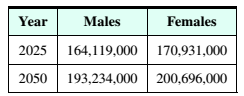Chapter 9, Problem 23REMathematical Excursions (MindTap C...

4th Edition
Richard N. Aufmann + 3 others
ISBN: 9781305965584

Solutions

Chapter
SectionMathematical Excursions (MindTap C...

4th Edition
Richard N. Aufmann + 3 others
ISBN: 9781305965584
Textbook Problem

Demographics According to the U.S. Bureau of the Census, the population of males and females in the United States in 2025 and 2050 is projected to be as shown in the table below.a. What percent of the projected population in 2025 is female? Round to the nearest tenth of a percent.b. Does the percent of the projected population that is female in 2050 differ by more or less than 1 % from the percent that is female in 2025 ?

a.

To determine

The percentage of female in projected population of 2025.

Explanation

Given information: The table shows the projected population of males and females in the United States in 2025 and 2050.

 Year Males Females 2025 164,119,000 170,931,000 2050 193,234,000 200,696,000

Calculatio...

b.

To determine

The percentage of change in female population of 2050 from 2025.

Still sussing out bartleby?

Check out a sample textbook solution.

See a sample solution

The Solution to Your Study Problems

Bartleby provides explanations to thousands of textbook problems written by our experts, many with advanced degrees!

Get Started

In Exercises 7-28, perform the indicated operations and simplify each expression. 10. 3x24xy4y2x2y(2yx)2x3y

Applied Calculus for the Managerial, Life, and Social Sciences: A Brief Approach

Find (f1)(a). f(x)=x3+4x+4,a=3

Calculus (MindTap Course List)

Expand each expression in Exercises 122. (x+yxy)2

Finite Mathematics and Applied Calculus (MindTap Course List)

The basic SI unit of time is ____.

Elementary Technical Mathematics

If sin=725, find cos and sec.

Elementary Geometry for College Students